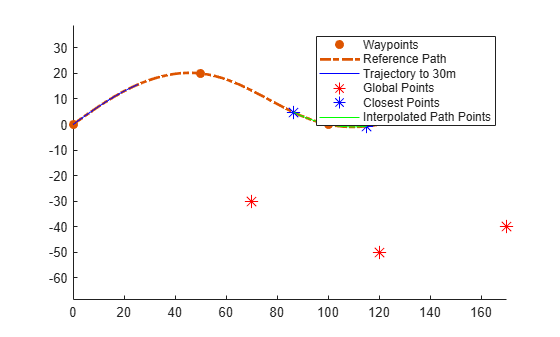# frenet2global

Convert Frenet states to global states

## Syntax

``globalState = frenet2global(refPath,frenetState)``
``globalState = frenet2global(refPath,frenetState,latTimeDerivatives)``

## Description

example

````globalState = frenet2global(refPath,frenetState)` converts Frenet trajectory states to global states.```
````globalState = frenet2global(refPath,frenetState,latTimeDerivatives)` accepts `latTimeDerivatives` containing 1st and 2nd order derivatives of lateral deviation with respect to time and a flag indicating if the heading should flip during the conversion to global coordinates.```

## Examples

collapse all

Generate a reference path from a set of waypoints.

```waypoints = [0 0; 50 20; 100 0; 150 10]; refPath = referencePathFrenet(waypoints);```

Create a trajectoryGeneratorFrenet object from the reference path.

`connector = trajectoryGeneratorFrenet(refPath);`

Generate a five-second trajectory between the path origin and a point 30 m down the path as Frenet states.

```initState = [0 0 0 0 0 0]; % [S dS ddS L dL ddL] termState = [30 0 0 0 0 0]; % [S dS ddS L dL ddL] frenetTraj = connect(connector,initState,termState,5);```

Convert the trajectory to the global states.

`globalTraj = frenet2global(refPath,frenetTraj.Trajectory);`

Display the reference path and the trajectory.

```show(refPath); axis equal hold on plot(globalTraj(:,1),globalTraj(:,2),'b')```

Specify global points and find the closest points on reference path.

```globalPoints = waypoints(2:end,:) + [20 -50]; nearestPathPoint = closestPoint(refPath,globalPoints);```

Display the global points and the closest points on reference path.

```plot(globalPoints(:,1),globalPoints(:,2),'r*','MarkerSize',10) plot(nearestPathPoint(:,1),nearestPathPoint(:,2),'b*','MarkerSize',10)```

Interpolate between the arc lengths of the first two closest points along the reference path.

```arclengths = linspace(nearestPathPoint(1,6),nearestPathPoint(2,6),10); pathStates = interpolate(refPath,arclengths);```

Display the interpolated path points.

```plot(pathStates(:,1),pathStates(:,2),'g') legend(["Waypoints","Reference Path","Trajectory to 30m",... "Global Points","Closest Points","Interpolated Path Points"])```## Input Arguments

collapse all

Reference path, specified as a `referencePathFrenet` object.

States in the Frenet coordinate frame, returned as a P-by-6 numeric matrix with rows of form `[S dS ddS L dL ddL]`, where `S` is the arc length and `L` is the perpendicular deviation from the direction of the reference path. Derivatives of `S` are relative to time. Derivatives of `L` are relative to the arc length, `S`. P is the total number of Frenet states.

Lateral time derivatives, specified as an N-by-3 matrix where each row is of the form [dL/dt ddL/dt^2 invertHeading] and N is the total number of points in `points`. Each row contains the 1st and 2nd order time derivatives of lateral deviation and a flag, invertHeading, which indicates whether the heading should be flipped when converting to global coordinates (`true`) or not (`false`).

Note

If defining `latTimeDerivatives` without the use of `global2frenet`, the following rules should be followed:

1. The invertHeading flag should be true when:

1. The vehicle is moving in reverse (speed is less than 0)

2. The vehicle is stationary (speed is equal to 0), and the vehicle is facing away from the path's tangent vector. i.e. cos(|tangentAngle(obj,S)-thetaExpected|) < 0

2. If 1b is true, then dL/dS must be negated.

## Output Arguments

collapse all

States in the global coordinate frame, specified as a P-by-6 numeric matrix with rows of form `[x y theta kappa speed accel]`, where:

• `x` `y` and `theta` –– SE(2) state expressed in global coordinates, with `x` and `y` in meters and `theta` in radians.

• `kappa` –– Curvature, or inverse of the radius, in meters.

• `speed` –– Speed in the `theta` direction in m/s.

• `accel` –– Acceleration in the `theta` direction in m/s2.

P is the total number of Global states.

## Version History

Introduced in R2020b